## Selina Concise Mathematics Class 10 ICSE Solutions Remainder and Factor Theorems

Selina Publishers Concise Mathematics Class 10 ICSE Solutions Chapter 8 Remainder and Factor Theorems

### Remainder and Factor Theorems Exercise 8A – Selina Concise Mathematics Class 10 ICSE Solutions

Question 1.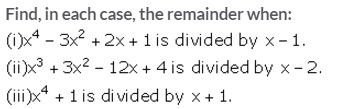Solution:
By remainder theorem we know that when a polynomial f (x) is divided by x – a, then the remainder is f(a).Question 2.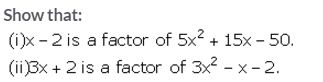Solution:
(x – a) is a factor of a polynomial f(x) if the remainder, when f(x) is divided by (x – a), is 0, i.e., if f(a) = 0.Question 3.
Use the Remainder Theorem to find which of the following is a factor of 2x3 + 3x2 – 5x – 6.
(i) x + 1
(ii) 2x – 1
(iii) x + 2
Solution:
By remainder theorem we know that when a polynomial f (x) is divided by x – a, then the remainder is f(a).
Let f(x) = 2x3 + 3x2 – 5x – 6
(i) f (-1) = 2(-1)3 + 3(-1)2 – 5(-1) – 6 = -2 + 3 + 5 – 6 = 0
Thus, (x + 1) is a factor of the polynomial f(x).Thus, (2x – 1) is not a factor of the polynomial f(x).
(iii) f (-2) = 2(-2)3 + 3(-2)2 – 5(-2) – 6 = -16 + 12 + 10 – 6 = 0
Thus, (x + 2) is a factor of the polynomial f(x).

Question 4.
(i) If 2x + 1 is a factor of 2x2 + ax – 3, find the value of a.
(ii) Find the value of k, if 3x – 4 is a factor of expression 3x2 + 2x – k.
Solution:Question 5.
Find the values of constants a and b when x – 2 and x + 3 both are the factors of expression x3 + ax2 + bx – 12.
Solution: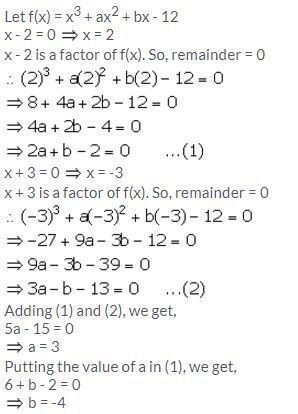Question 6.
find the value of k, if 2x + 1 is a factor of (3k + 2)x3 + (k – 1).
Solution:Question 7.
Find the value of a, if x – 2 is a factor of 2x5 – 6x4 – 2ax3 + 6ax2 + 4ax + 8.
Solution:
f(x) = 2x5 – 6x4 – 2ax3 + 6ax2 + 4ax + 8
x – 2 = 0 ⇒  x = 2
Since, x – 2 is a factor of f(x), remainder = 0.
2(2)5 – 6(2)4 – 2a(2)3 + 6a(2)2 + 4a(2) + 8 = 0
64 – 96 – 16a + 24a + 8a + 8 = 0
-24 + 16a = 0
16a = 24
a = 1.5

Question 8.
Find the values of m and n so that x – 1 and x + 2 both are factors of x3 + (3m + 1) x2 + nx – 18.
Solution:Question 9.
When x3 + 2x2 – kx + 4 is divided by x – 2, the remainder is k. Find the value of constant k.
Solution:Question 10.
Find the value of a, if the division of ax3 + 9x2 + 4x – 10 by x + 3 leaves a remainder 5.
Solution:Question 11.
If x3 + ax2 + bx + 6 has x – 2 as a factor and leaves a remainder 3 when divided by x – 3, find the values of a and b.
Solution:Question 12.
The expression 2x3 + ax2 + bx – 2 leaves remainder 7 and 0 when divided by 2x – 3 and x + 2 respectively. Calculate the values of a and b.
Solution:Question 13.
What number should be added to 3x3 – 5x2 + 6x so that when resulting polynomial is divided by x – 3, the remainder is 8?
Solution: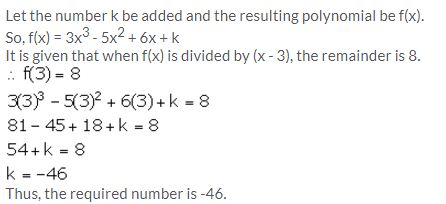Question 14.
What number should be subtracted from x3 + 3x2 – 8x + 14 so that on dividing it with x – 2, the remainder is 10.
Solution:Question 15.
The polynomials 2x3 – 7x2 + ax – 6 and x3 – 8x2 + (2a + 1)x – 16 leaves the same remainder when divided by x – 2. Find the value of ‘a’.
Solution: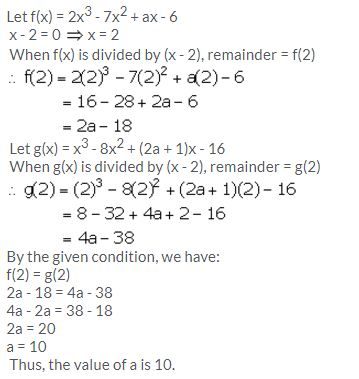Question 16.
If (x – 2) is a factor of the expression 2x3 + ax2 + bx – 14 and when the expression is divided by (x – 3), it leaves a remainder 52, find the values of a and b
Solution:Question 17.
Find ‘a‘ if the two polynomials ax3 + 3x2 – 9 and 2x3 + 4x + a, leave the same remainder when divided by x + 3.
Solution: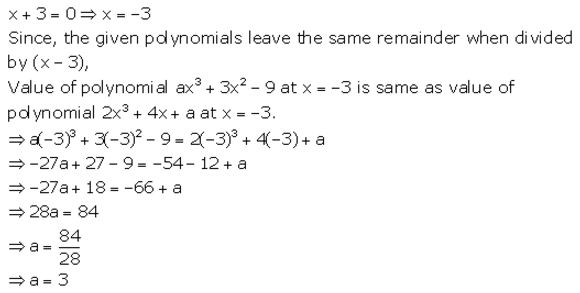### Remainder and Factor Theorems Exercise 8B – Selina Concise Mathematics Class 10 ICSE Solutions

Question 1.
Using the Factor Theorem, show that:
(i) (x – 2) is a factor of x3 – 2x2 – 9x + 18. Hence, factorise the expression x3 – 2x2 – 9x + 18 completely.
(ii) (x + 5) is a factor of 2x3 + 5x2 – 28x – 15. Hence, factorise the expression 2x3 + 5x2 – 28x – 15 completely.
(iii) (3x + 2) is a factor of 3x3 + 2x2 – 3x – 2. Hence, factorise the expression 3x3 + 2x2 – 3x – 2 completely.
Solution:Question 2.
Using the Remainder Theorem, factorise each of the following completely.
(i) 3x+ 2x2 − 19x + 6
(ii) 2x3 + x2 – 13x + 6
(iii) 3x3 + 2x2 – 23x – 30
(iv) 4x3 + 7x2 – 36x – 63
(v) x3 + x2 – 4x – 4
Solution:Question 3.
Using the Remainder Theorem, factorise the expression 3x3 + 10x2 + x – 6. Hence, solve the equation 3x3 + 10x2 + x – 6 = 0.
Solution: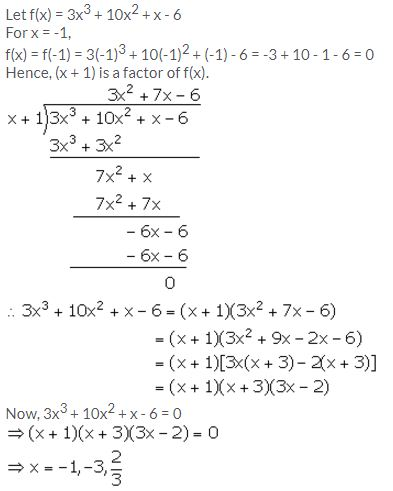Question 4.
Factorise the expression f (x) = 2x3 – 7x2 – 3x + 18. Hence, find all possible values of x for which f(x) = 0.
Solution: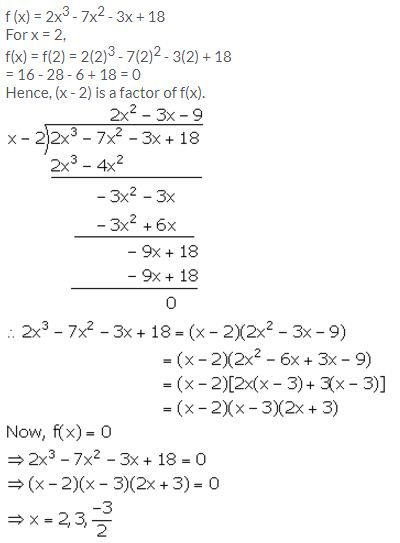Question 5.
Given that x – 2 and x + 1 are factors of f(x) = x3 + 3x2 + ax + b; calculate the values of a and b. Hence, find all the factors of f(x).
Solution: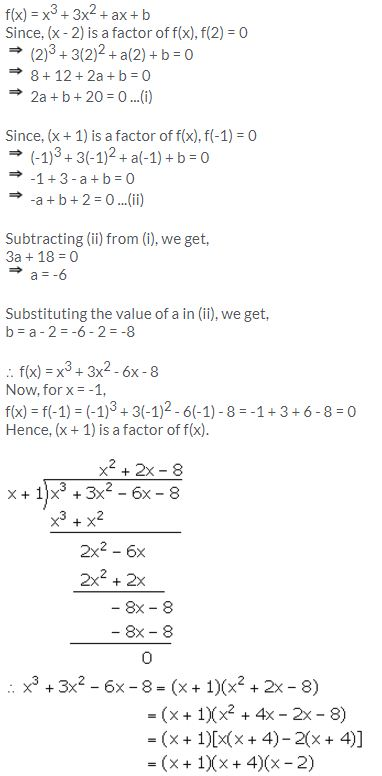Question 6.
The expression 4x3 – bx2 + x – c leaves remainders 0 and 30 when divided by x + 1 and 2x – 3 respectively. Calculate the values of b and c. Hence, factorise the expression completely.
Solution:Question 7.
If x + a is a common factor of expressions f(x) = x2 + px + q and g(x) = x2 + mx + n;Solution:Question 8.
The polynomials ax3 + 3x2 – 3 and 2x3 – 5x + a, when divided by x – 4, leave the same remainder in each case. Find the value of a.
Solution:
Let f(x) = ax3 + 3x2 – 3
When f(x) is divided by (x – 4), remainder = f(4)
f(4) = a(4)3 + 3(4)2 – 3 = 64a + 45
Let g(x) = 2x3 – 5x + a
When g(x) is divided by (x – 4), remainder = g(4)
g(4) = 2(4)3 – 5(4) + a = a + 108
It is given that f(4) = g(4)
64a + 45 = a + 108
63a = 63
a = 1

Question 9.
Find the value of ‘a’, if (x – a) is a factor of x3 – ax2 + x + 2.
Solution:
Let f(x) = x3 – ax2 + x + 2
It is given that (x – a) is a factor of f(x).
Remainder = f(a) = 0
a3 – a3 + a + 2 = 0
a + 2 = 0
a = -2

Question 10.
Find the number that must be subtracted from the polynomial 3y3 + y2 – 22y + 15, so that the resulting polynomial is completely divisible by y + 3.
Solution:
Let the number to be subtracted from the given polynomial be k.
Let f(y) = 3y3 + y2 – 22y + 15 – k
It is given that f(y) is divisible by (y + 3).
Remainder = f(-3) = 0
3(-3)3 + (-3)2 – 22(-3) + 15 – k = 0
-81 + 9 + 66 + 15 – k = 0
9 – k = 0
k = 9

### Remainder and Factor Theorems Exercise 8C – Selina Concise Mathematics Class 10 ICSE Solutions

Question 1.
Show that (x – 1) is a factor of x3 – 7x2 + 14x – 8. Hence, completely factorise the given expression.
Solution: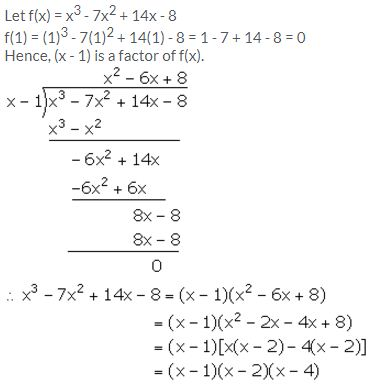Question 2.
Using Remainder Theorem, factorise:
x3 + 10x2 – 37x + 26 completely.
Solution:Question 3.
When x3 + 3x2 – mx + 4 is divided by x – 2, the remainder is m + 3. Find the value of m.
Solution:
Let f(x) = x3 + 3x2 – mx + 4
According to the given information,
f(2) = m + 3
(2)3 + 3(2)2 – m(2) + 4 = m + 3
8 + 12 – 2m + 4 = m + 3
24 – 3 = m + 2m
3m = 21
m = 7

Question 4.
What should be subtracted from 3x3 – 8x2 + 4x – 3, so that the resulting expression has x + 2 as a factor?
Solution:
Let the required number be k.
Let f(x) = 3x3 – 8x2 + 4x – 3 – k
According to the given information,
f (-2) = 0
3(-2)3 – 8(-2)2 + 4(-2) – 3 – k = 0
-24 – 32 – 8 – 3 – k = 0
-67 – k = 0
k = -67
Thus, the required number is -67.

Question 5.
If (x + 1) and (x – 2) are factors of x3 + (a + 1)x2 – (b – 2)x – 6, find the values of a and b. And then, factorise the given expression completely.
Solution:
Let f(x) = x3 + (a + 1)x2 – (b – 2)x – 6
Since, (x + 1) is a factor of f(x).
Remainder = f(-1) = 0
(-1)3 + (a + 1)(-1)2 – (b – 2) (-1) – 6 = 0
-1 + (a + 1) + (b – 2) – 6 = 0
a + b – 8 = 0 …(i)
Since, (x – 2) is a factor of f(x).
Remainder = f(2) = 0
(2)3 + (a + 1) (2)2 – (b – 2) (2) – 6 = 0
8 + 4a + 4 – 2b + 4 – 6 = 0
4a – 2b + 10 = 0
2a – b + 5 = 0 …(ii)
Adding (i) and (ii), we get,
3a – 3 = 0
a = 1
Substituting the value of a in (i), we get,
1 + b – 8 = 0
b = 7
f(x) = x3 + 2x2 – 5x – 6
Now, (x + 1) and (x – 2) are factors of f(x). Hence, (x + 1) (x – 2) = x2 – x – 2 is a factor of f(x).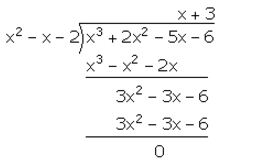f(x) = x3 + 2x2 – 5x – 6 = (x + 1) (x – 2) (x + 3)

Question 6.
If x – 2 is a factor of x2 + ax + b and a + b = 1, find the values of a and b.
Solution:
Let f(x) = x2 + ax + b
Since, (x – 2) is a factor of f(x).
Remainder = f(2) = 0
(2)2 + a(2) + b = 0
4 + 2a + b = 0
2a + b = -4 …(i)
It is given that:
a + b = 1 …(ii)
Subtracting (ii) from (i), we get,
a = -5
Substituting the value of a in (ii), we get,
b = 1 – (-5) = 6

Question 7.
Factorise x3 + 6x2 + 11x + 6 completely using factor theorem.
Solution: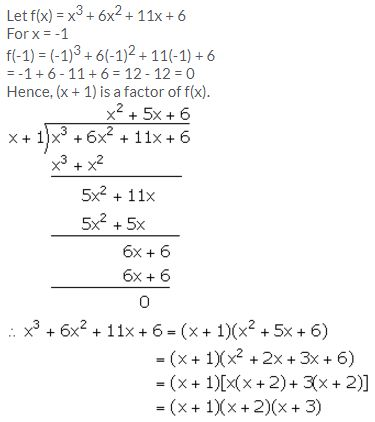Question 8.
Find the value of ‘m’, if mx3 + 2x2 – 3 and x2 – mx + 4 leave the same remainder when each is divided by x – 2.
Solution:
Let f(x) = mx3 + 2x2 – 3
g(x) = x2 – mx + 4
It is given that f(x) and g(x) leave the same remainder when divided by (x – 2). Therefore, we have:
f (2) = g (2)
m(2)3 + 2(2)2 – 3 = (2)2 – m(2) + 4
8m + 8 – 3 = 4 – 2m + 4
10m = 3
m = 3/10

Question 9.
The polynomial px3 + 4x2 – 3x + q is completely divisible by x2 – 1; find the values of p and q. Also, for these values of p and q factorize the given polynomial completely.
Solution:
Let f(x) = px3 + 4x2 – 3x + q
It is given that f(x) is completely divisible by (x2 – 1) = (x + 1)(x – 1).
Therefore, f(1) = 0 and f(-1) = 0
f(1) = p(1)3 + 4(1)2 – 3(1) + q = 0
p + q + 1 = 0 …(i)
f(-1) = p(-1)3 + 4(-1)2 – 3(-1) + q = 0
-p + q + 7 = 0 …(ii)
Adding (i) and (ii), we get,
2q + 8 = 0
q = -4
Substituting the value of q in (i), we get,
p = -q – 1 = 4 – 1 = 3
f(x) = 3x3 + 4x2 – 3x – 4
Given that f(x) is completely divisible by (x2 – 1).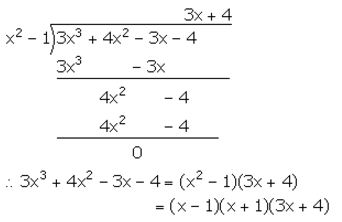Question 10.
Find the number which should be added to x2 + x + 3 so that the resulting polynomial is completely divisible by (x + 3).
Solution:
Let the required number be k.
Let f(x) = x2 + x + 3 + k
It is given that f(x) is divisible by (x + 3).
Remainder = 0
f (-3) = 0
(-3)2 + (-3) + 3 + k = 0
9 – 3 + 3 + k = 0
9 + k = 0
k = -9
Thus, the required number is -9.

Question 11.
When the polynomial x3 + 2x2 – 5ax – 7 is divided by (x – 1), the remainder is A and when the polynomial x3 + ax2 – 12x + 16 is divided by (x + 2), the remainder is B. Find the value of ‘a’ if 2A + B = 0.
Solution:
It is given that when the polynomial x3 + 2x2 – 5ax – 7 is divided by (x – 1), the remainder is A.
(1)3 + 2(1)2 – 5a(1) – 7 = A
1 + 2 – 5a – 7 = A
– 5a – 4 = A …(i)
It is also given that when the polynomial x3 + ax2 – 12x + 16 is divided by (x + 2), the remainder is B.
x3 + ax2 – 12x + 16 = B
(-2)3 + a(-2)2 – 12(-2) + 16 = B
-8 + 4a + 24 + 16 = B
4a + 32 = B …(ii)
It is also given that 2A + B = 0
Using (i) and (ii), we get,
2(-5a – 4) + 4a + 32 = 0
-10a – 8 + 4a + 32 = 0
-6a + 24 = 0
6a = 24
a = 4

Question 12.
(3x + 5) is a factor of the polynomial (a – 1)x3 + (a + 1)x2 – (2a + 1)x – 15. Find the value of ‘a’, factorise the given polynomial completely.
Solution:Question 13.
When divided by x – 3 the polynomials x3 – px2 + x + 6 and 2x3 – x2 – (p + 3) x – 6 leave the same remainder. Find the value of ‘p’.
Solution:
If (x – 3) divides f(x) = x3 – px2 + x + 6, then,
Remainder = f(3) = 33 – p(3)2 + 3 + 6 = 36 – 9p
If (x – 3) divides g(x) = 2x3 – x2 – (p + 3) x – 6, then
Remainder = g(3) = 2(3)3 – (3)2 – (p + 3) (3) – 6 = 30 – 3p
Now, f(3) = g(3)
⇒ 36 – 9p = 30 – 3p
⇒ -6p = -6
⇒  p = 1

Question 14.
Use the Remainder Theorem to factorise the following expression:
2x3 + x2 – 13x + 6
Solution: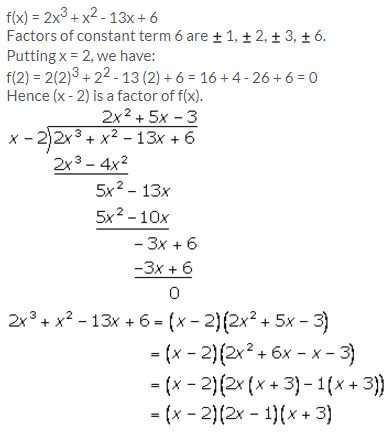Question 15.
Using remainder theorem, find the value of k if on dividing 2x3 + 3x2 – kx + 5 by x – 2, leaves a remainder 7.
Solution:
Let f(x) = 2x3 + 3x2 – kx + 5
Using Remainder Theorem, we have
f(2) = 7
∴ 2(2)3 + 3(2)2 – k(2) + 5 = 7
∴ 16 + 12 – 2k + 5 = 7
∴ 33 – 2k = 7
∴ 2k = 26
∴ k = 13

Question 16.
What must be subtracted from 16x3 – 8x2 + 4x + 7 so that the resulting expression has 2x + 1 as a factor?
Solution:
Here, f(x) = 16x3 – 8x2 + 4x + 7
Let the number subtracted be k from the given polynomial f(x).
Given that 2x + 1 is a factor of f(x).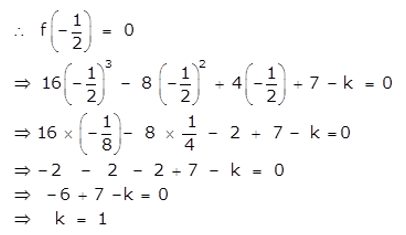Therefore 1 must be subtracted from 16x3 – 8x2 + 4x + 7 so that the resulting expression has 2x + 1 as a factor.

More Resources for Selina Concise Class 10 ICSE Solutions